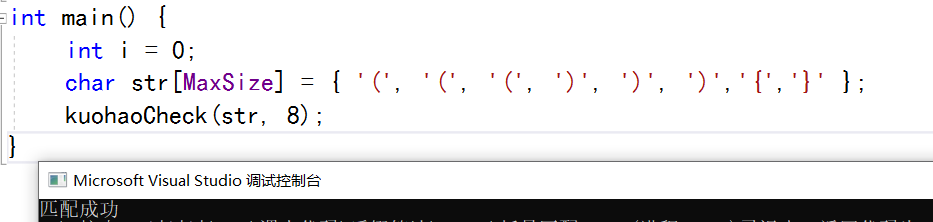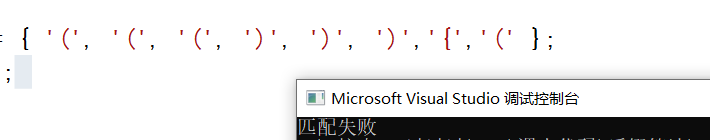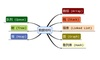• 括号匹配，栈和队列的应用 #include <stdio.h> #include <stdlib.h> #define true 1 #define false 0 #define ok 1 #define error 0 #define overflow -2 #define STACK_INIT_SIZE 100 #define ...

括号匹配，栈的应用

#include <stdio.h>
#include <stdlib.h>
#define true 1
#define false 0
#define ok 1
#define error 0
#define overflow -2
#define STACK_INIT_SIZE 100
#define STACKINCREMENT 10
typedef int status;
typedef struct {
char *base;
char *top;
int stacksize;
}sqstack;
status initstack(sqstack &s) {//初始化stack；
s.base = (char *)malloc(STACK_INIT_SIZE * sizeof(char));
if (!s.base) exit(overflow);
s.top = s.base;
s.stacksize = STACK_INIT_SIZE;
return ok;
}
status push(sqstack &s, char e) {
if (s.top - s.base >= s.stacksize) {
s.base = (char *)realloc(s.base, (s.stacksize + STACKINCREMENT) * sizeof(char));
s.top = s.base + s.stacksize;
s.stacksize += STACKINCREMENT;
}
*s.top++= e;
return ok;
}
status pop(sqstack &s,char &e) {
if (s.base == s.top) return error;
e = *--s.top;
return ok;
}
status stackempty(sqstack s) {
if (s.base == s.top) return true;
else return false;
}
status gettop(sqstack s, char &e) {
if (s.top == s.base) return error;
e = *(s.top - 1);
return ok;
}
void judge(){
sqstack S;
initstack(S);
bool flag=true;
char op, a, b;
printf("请输入你要判断的这些括号(只能是“（、）、[、]”)\n");
op = getchar();
while (op != '\n'&& flag==true) {
switch (op) {
case '[':
case '(':push(S, op); break;
case ')':gettop(S, a); if (a == '(') pop(S,b);
else flag = false; break;
case ']':gettop(S, a); if (a == '[') pop(S,b);
else flag = false; break;
default: break;
}
op = getchar();
}
if (stackempty(S)) flag = true;
else flag = false;
if (flag) printf("匹配正确！");
else printf("匹配错误！");
}
int main() {
judge();
return 0;
}

展开全文数据结构
• 数据结构中，括号匹配问题可以说是一个非常经典的问题。 【问题描述】假设一算术表达式中包括三种括号：圆括号“(”和“)”，方括号“[”和“]”，花括号“{”和“}”，且三种括号可按任意次序嵌套使用，试编写程序...

数据结构中，括号匹配问题可以说是一个非常经典的问题。

【问题描述】假设一算术表达式中包括三种括号：圆括号“(”和“)”，方括号“[”和“]”，花括号“{”和“}”，且三种括号可按任意次序嵌套使用，试编写程序判定输入的表达式所含的括号是否正确配对出现（提示：表达式可以存入一个数据元素为字符的顺序表/数组中）。若匹配，则输出“Y”，否则输出“N”以及从左侧第一个不匹配字符的位置（从0开始编号）。【输入形式】含括号的算术表达式

【输出形式】Y 或者N 左侧第一个不匹配字符的位置

【样例输入】3+(44*]5-{6*[7*(45-10)]}])

【样例输出】N6
【样例说明】判断括号是否匹配涉及两方面，括号个数和出现次序的判定

#include<stdio.h>
int main()
{
int a={0};
for(int l=0;l<1000;l++)
{
a[l]=0;
}
int i,j,c;
int m;//第一个偶数出现的位置
m=0;
i=0;
j=999;
//储存整数数组
do
{
scanf("%d",&c);
{if(c%2==1)
a[i++]=c;
if(c%2==0)
a[j--]=c;//这样子做会让第一个偶数放在最后
}
}while (getchar()==',');

//计算m
for(i=0;i<1000;i++)
{
if(a[i]%2==1||a[i]==0)
m++;
}
//printf("%d",m);
//printf("\n");

//改变偶数的位置
int n=m;//储存m原来的值
int x=1000-m;
int y=0;
int b[x];
//复制偶数数组
for(j=0;j<x;j++)
{
b[j]=a[m];
m++;
}
for(m=n;m<1000;m++)
{
a[m]=b[x-1-y];
y++;
}

//计算非0的个数
int notzero=0;
for(j=0;j<1000;j++)
{
if(a[j]!=0)
notzero++;
}

j=0;
for(int k=0;k<1000;k++)
{
if(a[k]==0)
continue;
else
{
printf("%d",a[k]);
if(j<notzero-1)
{
putchar(',');
j++;
}
}
}
return 0;
}

展开全文数据结构
• #include<stdio.h> #include<stdlib.h>...//新建一个栈结构 typedef struct stack{ ElementType a; struct stack *next; } List,*lp; //初始化栈 struct stack *create(); int Pus.
#include<stdio.h>
#include<stdlib.h>
#include <malloc.h>
#define MAX 1001

//此程序采用顺序栈做法

typedef char ElementType;

//新建一个栈结构
typedef struct stack{
ElementType a;
struct stack *next;
} List,*lp;

//初始化栈
struct stack *create();
int Push(lp L,ElementType x);//入栈
int Pop(lp L,ElementType e);//出栈

int main(){
char s[MAX];//新建一个数组并存储输入的代码
gets(s);
int i=0,left=0,right=0,flag=1 ;
lp L=NULL;//新建一个栈指针，并初始化
L=create();
while(s[i]!='\0'){
if(s[i]=='('){
Push(L,'(');
left++;
}	else if(s[i]=='['){
Push(L,'[');
left++;
} else if(s[i]=='{') {
Push(L,'{');
left++;
} else if(s[i]==')'){
if(Pop(L,'(')!=1)flag=0;
right++;
//printf("%d",flag);
}else if(s[i]==']') {
if(Pop(L,'[')!=1) flag=0 ;
right++;
//printf("%d",flag);
} else if(s[i]=='}') {
if(Pop(L,'{')!=1) flag=0 ;
right++;
//printf("%d",flag);
}
i++;

}

if(L->next!=NULL) {flag=0;
}
printf("%d %d\n",left,right);

if(flag==1){
printf("YES\n");
}else{
printf("NO\n");
}
}

struct stack *create(){
lp s=NULL;//新建一个栈指针
s=(lp)malloc(sizeof(lp));
s->next=NULL;
return s;
}

int Push(lp L,ElementType x){
lp p=(lp)malloc(sizeof(lp));
p->a=x;
p->next=L->next;
L->next=p;
return 1;
}

int Pop(lp L,ElementType e){
lp p=(lp)malloc(sizeof(lp));
if(L->next==NULL) return 0;
p=L->next;
if(p->a==e){
L->next=p->next;
free(p);
return 1;
}else{
return 0;
}
}

展开全文c语言 数据结构
• 王道408 数据结构括号匹配 由于不会用scanf和while来读写括号直接就定义字符数组了 #define MaxSize 50 //定义最大个数 #pragma warning(disable : 4996) #include <stdio.h> #include <stdlib.h> #...

王道408 数据结构括号匹配

由于不会用scanf和while来读写括号直接就定义字符数组了

#define MaxSize 50      //定义最大个数
#pragma warning(disable : 4996)
#include <stdio.h>
#include <stdlib.h>
#include <cmath>
typedef struct {
int data[MaxSize];  //存放栈中元素
int top;           //栈顶知指针
}SqStack;

// 初始化栈顶指针
void  InitStack(SqStack &S) {
S.top = -1;
}

//判断栈空
bool StackEmpty(SqStack S) {
if (S.top == -1) {//栈空
return true;
}
else {           //不空
return false;
}
}

//进栈
bool Push(SqStack &S, char x) {
if (S.top == MaxSize - 1) {//栈满报错
return false;
}
S.data[++S.top] = x;     //指针+1，在入栈
//等价写法
//S.top=S.top+1
//S.data[S.top] = x
return true;
}

//出栈
bool Pop(SqStack &S, char &x) {
if (S.top == -1) {    // 栈空报错
return false;
}
x = S.data[S.top--];//先出栈，指针再-1
return true;
}

//获得栈顶元素
bool GetTop(SqStack &S, char &x) {
if (S.top == -1) {   //栈空报错
return false;
}
x = S.data[S.top];//x记录栈顶元素
return true;
}

bool kuohaoCheck(char str[], int length) {
SqStack S;
InitStack(S);//初始化一个栈
for (int i = 0;i < length;i++) {
if (str[i] == '(' || str[i] == '[' || str[i] == '{') {
Push(S, str[i]);      //扫描左括号，入栈
}
else {
if (StackEmpty(S)) {// 扫描到右括号，且当前为栈空
printf("匹配失败");
return false;
}
char topElem;
Pop(S, topElem);//栈顶元素出栈
if (str[i] == '('&&topElem != ')') {//判断括号是否相等
printf("匹配失败");
return false;
}
if (str[i] == '{'&&topElem != '}') {
printf("匹配失败");
return false;
}
if (str[i] == '['&&topElem != ']') {
printf("匹配失败");
return false;
}
}

}
if (StackEmpty(S)) {
printf("匹配成功");
}
else {
printf("匹配失败");
}
//所有括号匹配完成后，栈空说明成功
}
int main() {
int i = 0;
char str[MaxSize] = { '(', '(', '(', ')', ')', ')','{','}' };
kuohaoCheck(str, 8);
}

运行结果展开全文数据结构
• 对于这个问题，可以使用栈的数据结构来解决，栈的定义在下方class Stack： 然后考虑匹配的算法，由于我们输入的第一个一定是左括号（如果是右括号则一定无法匹配），我们可以将输入的字符串中的左括号都压入栈中，...python 数据结构 算法
• 检验括号匹配的方法，就是对给定的字符串依次检验：若是左括号，入栈；若是右括 号，出栈一个左括号判断是否与之相匹配；是其它字符，不检验。检验到字符串尾，还要 检查栈是否空。只有栈空，整个字符串才匹配完。 ...数据结构 c语言
•数据结构
• 输入一串括号字符串，从左往右扫描，若遇到左括号则入栈，若遇到右括号则从栈顶出栈一个括号(肯定是左括号),然后与其匹配。 代码实现 #include<iostream> #include<string> using namespace std; const ...
• 栈的在实际生活中有着很多的应用，如括号匹配、进制转换、迷宫求解、递归、函数或过程的调用等；队列在生活中也有着很多的应用，如打印缓冲区数据、CPU处理请求的顺序等。此题要介绍的是栈的应用中的括号匹配问题。 ...数据结构 算法
• 大家也可以自己把所有的重要的数据结构的代码写出来保存起来，到时候就可以拿出来直接用啦~ 然后这个地方我使用了函数封装起来这一项功能，这样在主函数就可以直接调用啦~ 主要理解的突破点就是：如果匹配不成功，...
• 向程序中输入一连串括号组成的字符串，只有每个左括号匹配到和他成对的右括号的时候，程序才会输出True,否则就输出False. 示例： 输入:[([])][] 输出:True 输入:()[[]) 输出:False 算法分析 该题使用栈来进行数据...数据结构 c语言 算法
• 数据结构与算法》课程设计 40、括号匹配的检验 问题描述： 假设表达式中允许有两种括号：圆括号和方括号，其嵌套的顺序随意，即（（）[ ]）或[（[ ] [ ]）]等为正确格式，[（ ]）或（（（]均为不正确的格式。检验...数据结构 算法 括号匹配的检验 c++
• 用顺序栈实现括号匹配 算法思想如下： #include<stdio.h> #include<stdbool.h> #define MAXSIZE 10 typedef struct StackArr { char data[MAXSIZE]; int top; }Stack,*PSTACK; //函数声明 void ...数据结构 c语言
• 括号匹配算法该算法的原理：算法的内容：与该算法配套的压栈和弹栈算法以及结构体定义： 该算法的原理： 判断是否出现 {【（，出现则压入栈中，如果遇到}】），则弹出上一个栈与其对比。 算法的内容： void ...数据结构 c++ 算法 字符串
• 这只是其中一个例题，看完我对这道题的分析之后，关于括号匹配的问题你肯定能够掌握个差不多。转载请注明本文链接！ 目录 一、问题描述 二、问题分析 三、代码实现 （一）、定义结构体 （二）、初始...数据结构 c语言 开发语言
• /* 其它字符不处理，指针向后移 */ } if(StackEmpty(s)) /* 字符串结束时栈空 */ printf("括号匹配n"); else printf("缺乏右括号n"); } } int main(int argc, char* argv[]) { check();//括号匹配 return 0; }
• 本题要求通过栈来判断括号匹配问题，如果匹配输出yes!，否则输出no! 函数接口定义： void BracketMatch(LinkStack top) 其中，top是栈顶 裁判测试程序样例： #include <stdio.h> #include <stdlib.h...数据结构 c语言
• 括号匹配问题 星期一遇到一个括号匹配题，做了两天，给我整吐了，由于只有样例 {{{))) 这是括号不匹配。 题中所说有4种情况：右括号多，左括号多，匹配，不匹配。 我以为 )() 是右括号多， )( 是不匹配的情况，从此...
• ![在这里插入图片描述]...代码实现 //最后出现的左括号最先被匹配，用栈的特性， //遇到左括号就入栈，遇到右括号就.数据结构 c语言
• 用顺序栈解决括号匹配问题 测试代码 #include <stdio.h> #include <string.h> #define MaxStackSize 100 typedef char DataType; #include "SeqStack.h" void ExpIsCorrect(char exp[], int n){ //...
• } //括号匹配算法 status Parenthesis_match(sqstack *s,char *str) { int i=0, flag=0; SElemtype e; while(str[i] != '\0') { switch(str[i]) { case '(': push(s,str[i]); break; case '[': push(s,str[i]); ...
• 用栈实现检验括号匹配的算法没啥具体描述，数据结构的知识，急用，有重赏思想是 先进栈，获取第一个半边括号，标记一下，继续进栈直到获取到第二个与之匹配的另一外括号，然后出栈，取出内容。就这样。。数据结构 ...c语言括号匹配的检验
• 算法数据结构面试分享 符号匹配问题今天在帖子上看见有同学在问，如果一个字符串中包含大括号和小括号，我们该如何解决括号匹配问题。我们今天就一起看下这道题吧。按照我们之前的套路，按部就班来：1. 确保我们理解...
• 给定一串字符，不超过100个字符，可能包括括号、数字、字母、标点符号、空格，编程检查这一串字符中的( ) ,[ ],{ }是否匹配。输入格式:输入在一行中给出一行字符串，不超过100个字符，可能包括括号、数字、字母、...
• 一、问题描述： 设计算法检测表达式的括号是否匹配 注：包括 () [] {} 二、解题思路： 栈的应用 例如： ...当遇到右括号 )]} 时，将栈中的字符出栈并与此右括号比较，若此括号匹配，即 当右括号为数据结构 算法
• // 括号匹配函数 int match(char x,char y) { if((x=='('&& y==')')||(x=='['&& y==']')||(x=='{'&& y=='}')) return 1; else return 0; } int bracket(char *str) { SeqStack *S; ...数据结构 算法
• 就只有是() 就是先算内括号 在算外括号你说的这个问题是非常麻烦的如果你能看懂下面的代码的话 你就可以明白了如果你还是不明白，你就说一声 我会在给你解释的详细一些的\*下面这个程序是我原来学数据结构的时候...
• 栈的应用——括号匹配的检验 //栈的应用——括号匹配的检验 /*算法内容： 以此扫描所有字符，遇到左括号入栈，遇到右括号则弹出栈顶元素检查是否匹配。 匹配失败的情况： 1.左括号单 2.右括号单 3.左右括号不...算法 数据结构 c语言
•数据结构 算法 c语言
• 每一个左括号必须和相同类型的右括号匹配 左括号必须以正确的顺序闭合 空字符串也认为是成功匹配的字符串 输入：一个只包含上述六种字符的字符串 输出：括号匹配输出1，否则输出0 算法思路： 用gets_s()函数获取...数据结构 算法 字符串...

数据结构括号匹配数据结构 订阅